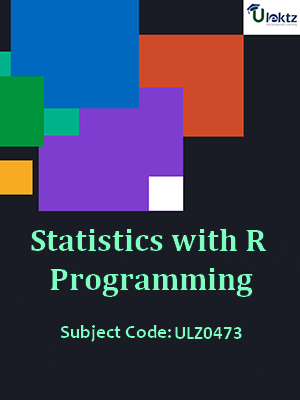•My WalletMy Order
•My Profile
•My Connections
•My Books
•My Videos
•My Tests
•My Calender
•My Messages
•My Shopping Cart
•My Orders
•Account Settings
•Help

# Book Details# Statistics With r Programming

 Course Code : ULZ0473 Author : uLektz University : General for All University Regulation : 2016 Categories : Computer Science Format :ePUB3 (DRM Protected) Type : eBook

FREE

Description :Statistics With r Programming of ULZ0473 covers the latest syllabus prescribed by General for All University for regulation 2016. Author: uLektz, Published by uLektz Learning Solutions Private Limited.

Note : No printed book. Only ebook. Access eBook using uLektz apps for Android, iOS and Windows Desktop PC.

##### Topics
###### UNIT-I INTRODUCTION TO R PROGRAMMING

1.1 Introduction, How to run R, R Sessions and Functions

1.2 Basic Math, Variables, Data Types, Vectors, Conclusion

1.3 Advanced data structures, data frames, lists, matrices, arrays, classes

###### UNIT-II R PROGRAMMING - STRUCTURES, CONTROL STATEMENTS & LOOPS

2.1 R Programming Structures, Control Statements, Loops - Looping Over Nonvector Sets, If-Else, Arithmetic and Boolean Operators and values, Default Values for Argument

2.2 Return Values, Deciding whether to explicitly call return- Returning Complex Objects, Functions are Objective, No Pointers in R

2.3 Recursion, A Quicksort Implementation-Extended Example: A Binary Search Tree

###### UNIT-III MATH FUNCTION AND SIMULATION IN R

3.1 Doing Math and Simulation in R, Math Function, Extended Example, Calculating Probability-Cumulative Sums and Products-Minima and Maxima- Calculus

3.2 Functions for Statistical Distributions, Sorting

3.3 Linear Algebra Operations on Vectors and Matrices, Extended Example: Vector cross Product- Extended Example: Finding Stationary Distribution of Markov Chains,

3.4 Set Operations

3.5 Input /output, Accessing the Keyboard and Monitor, Reading and writer Files

###### UNIT-IV GRAPHICS

4.1 Graphics, Creating a Graph, The Workhorse of R Base Graphics, the plot() Function

4.2 Customizing Graphs

4.3 Saving Graphs to Files

###### UNIT-V DISTRIBUTIONS

5.1 Probability Distributions, Normal Distribution-Binomial Distribution- Poisson Distributions-Other Distribution

5.2 Basic Statistics, Correlation and Covariance

5.3 T test, ANOVA

###### UNIT-VI LINEAR MODELS AND REGRESSION

6.1 Linear Models - Simple Linear Regression, , Multiple Regression and Generalized Linear Models, Logistic Regression, Poisson Regression-Other Generalized Linear Models

6.2 Survival analysis, Nonlinear Models, Splines, Decision- Random Forests